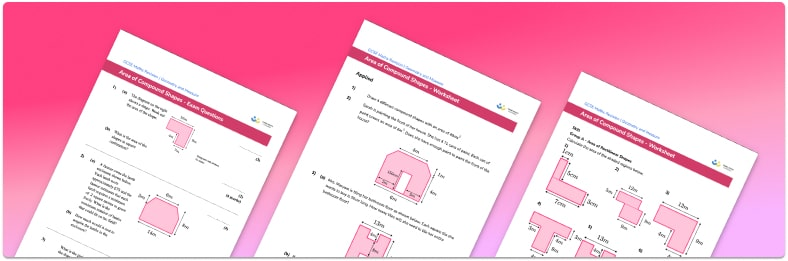# Area Of Compound Shapes Worksheet• Section 1 of the area of compound shapes worksheet contains 15 skills-based area of compound shapes questions, in 3 groups to support differentiation
• Section 2 contains 4 applied area of compound shapes questions with a mix of worded problems and deeper problem solving questions
• Section 3 contains 4 foundation and higher level GCSE exam style area of compound shapes questions
• Answers and a mark scheme for all questions are provided
• Questions follow variation theory with plenty of opportunities for students to work independently at their own level
• All questions created by fully qualified expert secondary maths teachers
• Suitable for GCSE maths revision for AQA, OCR and Edexcel exam board

• This field is for validation purposes and should be left unchanged.

You can unsubscribe at any time (each email we send will contain an easy way to unsubscribe). To find out more about how we use your data, see our privacy policy.

### Area of compound shapes at a glance

A compound shape is made from two or more simple shapes. Area is the amount of space covered by a 2D shape and is measured in square units – metric units of area include mm²,  cm² and m². To find the area of compound shapes, we need to break the shape into the component parts and find the area of each part. Sometimes the terminology ‘area of composite shapes’ is used which means exactly the same thing.

Rectilinear shapes are shapes with straight sides and right angles – i.e. made from rectangles. To find the area of a rectilinear shape, we need to find the area of each rectangle that makes up the shape, then find the sum of them in order to find the total area.

Other compound shapes can be made using simple 2D shapes – for example, a triangle placed on top of a parallelogram. Use the standard area formulae to calculate the area of each simple shape, then sum them to find the total area. In some problems, students may be required instead to use subtraction to find the area left when one shape is removed from another. This is particularly common in exam style questions and word problems – for example, finding the area of grass in a rectangular garden with a circular pond.

In addition to whole-number lengths and areas, it is important that students are confident working with decimals, particularly rounding an answer to a given number of decimal places.

Looking forward, students can then progress to additional area worksheets and other geometry worksheets, for example an angles in polygons worksheet or area and circumference of a circle worksheet.For more teaching and learning support on Geometry our GCSE maths lessons provide step by step support for all GCSE maths concepts.

## Do you have KS4 students who need more focused attention to succeed at GCSE?There will be students in your class who require individual attention to help them succeed in their maths GCSEs. In a class of 30, it’s not always easy to provide.

Help your students feel confident with exam-style questions and the strategies they’ll need to answer them correctly with our dedicated GCSE maths revision programme.

Lessons are selected to provide support where each student needs it most, and specially-trained GCSE maths tutors adapt the pitch and pace of each lesson. This ensures a personalised revision programme that raises grades and boosts confidence.

Find out more